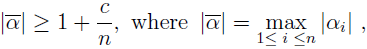# On some application of the spectral properties of the matrices

Aleksander Grytczuk and Izabela Kurzydło
Notes on Number Theory and Discrete Mathematics, ISSN 1310-5132
Volume 17, 2011, Number 2, Page 12—17

## Details

### Authors and affiliations

Aleksander GrytczukFaculty of Mathematics, Computer Science and Econometrics,
University of Zielona Gora,
65-516 Zielona Gora, Poland

Izabela KurzydłoFaculty of Mathematics, Computer Science and Econometrics,
University of Zielona Gora,
65-516 Zielona Gora, Poland

### Abstract

In the paper  A. Schinzel and H. Zassenhaus posed the following conjecture: If α ≠ 0 is an algebraic integer of degree n which is not a root of unity, then there exists a constant c > 0 such thatwhere α = α1; and α2, …, αn are the conjugates of α.
In this paper we give some information concerning this conjecture. In the proofs of the theorems we use some spectral properties of matrices.

### Keywords

• Conjecture of A. Schinzel and H. Zassenhaus
• Spectral properties of matrices

• 11R04
• 15A42

### References

1. P. E. Blanksby, H. L. Montgomery, Algebraic integers near the unit circle, Acta Arith. 18 (1971) 355-369.
2. D. W. Boyd, The maximal modulus of an algebraic integer, Math. Comp. 45 (1985) 243-249.
3. D. C. Cantor, E. G. Straus, On a conjecture of D. H. Lehmer, ibid. 42 (1982) 97-100.
4. E. Dobrowolski, On the maximal modulus of conjugates of an algebraic integer, Bull. Acad. Polon. Sci. Ser. Sci. Math. Astronom. Phys. 26 (1978) 291-292.
5. E. Dobrowolski, On a question of Lehmer and the number of irreducible factors of a polynomial, Acta Arith. 34 (1979) 391-401.
6. A. Dubickas, On a conjecture of A. Schinzel and H. Zassenhaus, Acta Arith. 63 (1993) 15-20.
7. A. Grytczuk, M. Szalkowski, Spectral properties of some matrices, Acta Acad. Pead. Agriensis Sectio Math. 20 (1991) 43-50.
8. P. Lankaster, Theory of matrices, Izd. Nauka, Moskwa (1978)(in Russian).
9. D. H. Lehmer, Factorization of certain cyclotomic functions, Ann. of Math. 34 (1933) 461-479.
10. R. Louboutin, Sur la mesure de Mahler d’un nombre algebrique, C. R. Acad. Sci. Paris 296 (1983) 707-708.
11. E. M. Matveev, On the cardinality of algebraic integers, Mat. Zametki 49 (1991) 152-154 (in Russian).
12. W. Narkiewicz, Elementary and analytic theory of algebraic numbers, PWN – Polish Scientific Publishers, Warszawa (1990).
13. G. Rhin, Q.Wu, On the smallest value of the maximal modulus of an algebraic integer, Math. Comp. 76 (2007) 1025-1038.
14. A. Schinzel, H. Zassenhaus, A refinement of two theorems of Kronecker, Michigan Math. J. 12 (1965) 81-85.
15. L. Stewart, Algebraic integers whose conjugates lie near the unit circle, Bull. Soc. Math. France 196 (1978) 169-176.

## Cite this paper

Grytczuk, A., & Kurzydło, I. (2011). On some application of the spectral properties of the matrices. Notes on Number Theory and Discrete Mathematics, 17(2), 12-17.